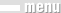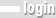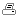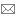Click here for login. Online visitors: 29M.Sc. In Air Transport Management Program  › Courses  › Quantitative Methods and Decision AnalysisQuantitative Methods and Decision Analysis
Course Description

This course is designed to teach such topics as:
Data Presentation and Summary, Probability Theory, Normal Distribution, Random Sampling and Sampling Distributions, Point and Interval Estimation, Hypothesis Testing, Comparing Two Samples, Simple Linear Regression, Multiple Linear Regression

Course Objectives

1. To develop an understanding of some basic statistical concepts
2. To use simple statistical techniques to analyze real world data and make business decisions
3. To become an informed and critical reader of quantitative arguments appeared in media and research reports

Course Outcomes

1. To view statistics not as merely mathematics but instead a process that consists of collecting business information, performing statistical analysis, and making business decision
2. To be proficient in some of the techniques used in statistical data collection, analysis and reporting
3. To conduct hypothesis testing using appropriate statistical tests
4. To build an appropriate multiple regression model to analyze business data and interpret its results
5. To utilize software such as Minitab for statistical analysis

Course Plan

1. Introduction-Fundamental Concepts in Data Analysis
2. Summarizing Data
3. A First Look at Probability
4. Random Variables and Probability Distributions
5. Some Special Probability Distributions
6. Random Sampling and Sampling Distributions
7. Point and Interval Estimation
8. Hypothesis Testing I
9. Hypothesis Testing II
10. Comparing Two Samples
11. Linear Regression and Correlation Methods I
12. Linear Regression and Correlation Methods II
13. Multiple Regression Methods I
14. Multiple Regression Methods II